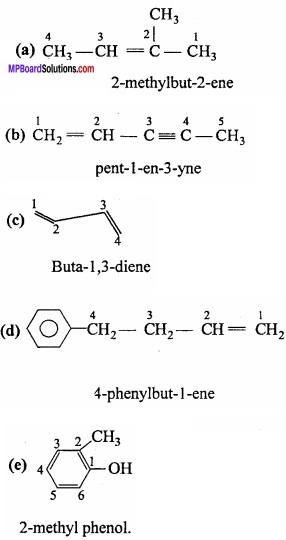# MP Board Class 11th Chemistry Important Questions Chapter 12 Organic Chemistry: Some Basic Principles and Techniques

## MP Board Class 11th Chemistry Important Questions Chapter 12 Organic Chemistry: Some Basic Principles and Techniques

### Organic Chemistry: Some Basic Principles and Techniques

Organic Chemistry: Some Basic Principles and Techniques Objective Type Questions

Question 1.

Question 1.
Aniline is generally purified by:
(a) Steam distillation
(b) Simple distillation
(c) Distillation under reduced presence
(d) Sublimation
(a) Steam distillation

Question 2.
Glycerol boils at 290°C with slight decomposition. Impure glycerol is purified by:
(a) Steam distillation
(b) Simple distillation
(c) Vacuum distillation
(d) Extraction with solvent
(c) Vacuum distillation

Question 3.
The blue or green colour obtained in Lassaigne’s test is due to the formation of:
(a) NaCN
(b) Na4[Fe(CN)6]4
(c) Fe3[Fe(CN)6]4
(d) Fe4[Fe(CN)0]3
(d) Fe4[Fe(CN)0]3Question 4.
In qualitative analysis of organic compound by Lassaigne’s test the violet colour obtained with sodium nitroprusside indicate the presence of:
(a) Nitrogen
(b) Sulphur
(c) Oxygen
(d) Halogen.
(b) Sulphur

Question 5.
The blood red colour compound formed during the qualitative analysis of nitrogen and sulphur together is:
(a) Fe4[Fe(CN)6]2
(b) Fe(SCN)3
(c) KSCN
(d) Na2S.NaCN.
(b) Fe(SCN)3

Question 6.
Kjeldahl’s as method is used for estimation of:
(a) Sulphur
(b) Netrogen
(c) Halogen
(d) Oxygen
(b) NetrogenQuestion 7.
A compound with empirical formula C2H5O had molecular mass 90. The formula of compound is:
(a) C4H10O2
(b) C2H5O
(c) C3H6O3
(d) C5H14O
(a) C4H10O2

Question 8.
The amount of sulphur present in an organic compound is estimated by changing into:
(a) H2S
(b) SO2
(c) H2SO4
(d) H2SO4
(d) H2SO4

Question 9.
The reagent used in Carius method to estimate halogen is:
(a) HNO3 and HCl
(b) HNO3 and H2SO4
(c) Fuming HNO3 and BaCl2
(d) Fuming HNO3 and AgNO3
(d) Fuming HNO3 and AgNO3

Question 10.
The gas collected in Duma’s method to estimate of nitrogen in organic compound is:
(a) N2
(b) NO
(c) NH3
(d) None of these
(a) N2Question 11.
An organic compound contain C = 80% and H = 20%. The compound shall be:
(a) C6H6
(b) C2H5 – OH
(c) C2H6
(d) CHCl3
(c) C2H6

Question 12.
An organic compound contain C = 39-9%, H = 6‘7% and O = 53.4%. The graphical formula shall be:
(a) CHO
(b) CHO2
(c) CH2O2
(d) CH2O
(d) CH2O

Question 13.
In an organic compound the ratio of mass is C:H:O = 4:1:5. Its empirical formula shall be:
(a) C2HO
(b) C2H4O4
(c) CH4O2
(d) CH3O
(d) CH3O

Question 14.
The main source of organic compound is:
(a) Coaltar
(b) Petroleum
(c) Both
(d) None of these
(c) BothQuestion 15.
But – 1,2 diene contains:
(a) Only sp – hybridized carbon atom
(b) Only sp2 – hybridized carbon atom
(c) sp and sp2 hybridized carbon atom
(d) sp, sp2 and sp3 hybridized carbon atom
(d) sp, sp2 and sp3 hybridized carbon atom

Question 16.
I.U.P.A.C. name of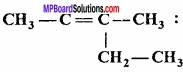(a) 2 – Ethyibut – 2ene
(b) 3 – Ethylbut – 2 – ane
(c) 2 – Methylpent – 3 – ene
(d) 3 – Methylpent – 2 – ene
(d) 3 – Methylpent – 2 – ene

Question 17.
The I.U.P.A.C. name of the compound having the formula of Cl3 – CCH2CHO is:
(a) 3,3,3 – trichloropropan – l – al
(b) 1,1,1 – trichloropropan – l – al
(c) 2,2,2 – trichloropropan – 1 – al
(d) Chloral.
(a) 3,3,3 – trichloropropan – l – al

Question 2.
Fill in the blanks:

1. Paper chromatography is based on the law of …………………………….
2. Column chromatography is based on the law of …………………………….
3. Aniline is purified by ………………………… method.
4. Hybridisation of central carbon atom of a carbocation is ……………………………..
5. Benzoic acid is purified by …………………………….
6. Before test of halogen, sodium extract is heated with ……………………………
7. Isomerism found in organic compounds of same series is ………………………………..
8. Chemical name of freon organic compound which is used in air conditions and refrigerators is ………………………….. and its chemical formula is ……………………..
9. ……………………….. ratio of elements in a compound is called its empirical formula.
10. The process of fractional crystallization of separation of two substances depending on the difference of ……………………………….
11. In Lassaigne’s test, blue or green colour is due to the formation of ……………………………….
12. On adding FeCl3 solution to sodium extract ……………………………. colour is obtained. The name of the compound is …………………………..
13. In organic compound, presence of amount of halogen can be detected by converting it into …………………………………….
14. A compound contain 80% carbon and 20% hydrogen, its formula will be ……………………………………
15. …………………………. gas is produced by the action of water on calcium carbide.
16. R – CONH2 is an ……………………………
17. Marsh gas mainly contain …………………………….. gas.

1. Distribution
3. Steam distillation
4. Sp2
5. Sublimation
6. Cone. HNO3,
7. Metamerism
8. Difluorodichloro methane CF2Cl2
9. Simplest
10. Solvent
11. Ferri – ferro cyanide
12. Red, ferric sulphocyanite
13. Silver halide
14. C2H6
15. Acetylene
16. Amide
17. Methane.Question 3.

1. Method used for separation of components on the basis of adsorption is known as?
2. What is conversion of solid substance on heating into vapours without changing into liquid known as?
3. Which element is detected by Duma’s method?
4. What is method of obtaining pure substance by vaporisation of impure liquid followed by condensation of vapours known as?
5. What is the charge on carbon in carbanion?
6. Which formula represents ratio of atoms of elements present in a molecule of a substance?
7. What is the nature of nucleophile?
8. What are cations carrying positive charge on carbon known as?
9. What is the nature of electrophile?
10. Which effect is responsible for the displacement of electrons of covalent bond towards or aways from carbon atom in an organic molecule?
11. Mixture of KMnO4 and KOH is known as?

1. Chromatography
2. Sublimation
3. Nitrogen
4. Distillation
5. Negative
6. Empirical formula
7. Electronegative
8. Carbocation
9. Electropositive
10. Inductive effect
11. Baeyer’s reagent.Question 4.
Match the following:
[I]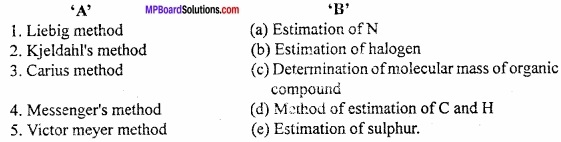1. (d)
2. (a)
3. (b)
4. (e)
5. (c)

[II]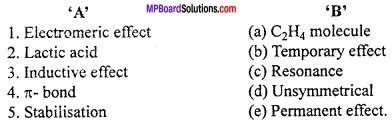1. (b)
2. (d)
3. (e)
4. (a)
5. (c)

[III]1. (a)
2. (d)
3. (b)
4. (c)

Organic Chemistry: Some Basic Principles and Techniques Very Short Answer Type Questions

Question 1.
What is the chemical name of CH3 – CH2 – CHCl – CH3?
iso – butyl chloride.

Question 2.
What is the mixture of KMnO4 and KOH called?
Bayer’s reagent.

Question 3.
IUPAC name of Vinegar?
Ethanoic acid.

Question 4.
What is the IUPAC name of grain alcohol?
Ethanol.Question 5.
For the mixture of CuSO4 and Camphor, Camphor is separated by?
Sublimation.

Question 6.
How purification of naphthalene was done?
By sublimation.

Question 7.
The purification by Column chromatography occur because?
Different absorption.

Question 8.
Purification of petroleum?
Fractional distillation.

Question 9.
Balsentein test performed for?
In halogen detection.Question 10.
Homolytic fission.

Question 11.
Main source of organic compounds are?
Coaltar and petroleum.

Question 12.
General formula of alcohol is?
CnH2n+1OH.

Question 13.
What is Chiral molecule?
Those which are not superimposable on their mirror images.

Question 14.
What is the name of the compound Cl – CH2 – CH2 – COOH?
3 – Chloro propanoic acid.

Question 15.
Write the structural formula of iso – butyl chloride?
CH3CH2CHClCH3.

Question 16.
CnH2n-2 is formula of?
Alkynes.Question 17.
What is Bayer’s reagent?
Alkaline KMnO4.

Question 18.
Compounds different in configuration are called?
Stereo isomers.

Question 19.
What is the IUPAC name of Cl3C.CH2CHO?
3,3,3 – trichloro propanol.

Question 20.
Which type of isomerism is found in nitro ethane?
Tautomerism.

Question 21.
Write the name and formula of Freon?
Difluoro – dichloro methane (CF2Cl2).

Question 22.
The mixture of o – nitrophenol and p – nitrophenol is separated by which method?
Vapour distillation method.

Question 23.
Which gas is present in Marsh gas?
Methane.

Question 24.
The decomposition of glycerine occurs before its b.p., by which method it can be purified?
Low pressure distillation.Question 25.
Formalin is formed by which compound?
HCHO.

Question 26.
What is the structural formula of gem – dihalide?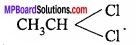Question 27.
What is the mechanism of this reaction:
CH3CH2I + KOH(aq) → CH3CH2OH + KI.
Nucleophilic substitution.

Question 28.
Kjeldahl’s method is used for estimation of which element?
Nitrogen.

Question 29.
What is Elution ?
The process of separation of products by different absorption rate is called elution

Question 30.
Which is the latest and better technique for the separation and purification of organic compounds?
Chromatography method.

Organic Chemistry: Some Basic Principles and Techniques Short Answer Type Questions

Question 1.
Why is it necessary to use acetic acid and not sulphuric acid for acidification of sodium extract for testing sulphur by lead acetate test?
For testing sulphur, the sodium extract is acidified with acetic acid because lead acetate is soluble and does not interfere with the test. If H2SO4 were used, lead acetate itself will react with H2SO4 to form white ppt. of lead sulphate which interfere the test.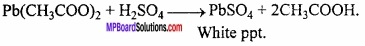Question 2.
Why is a solution of potassium hydroxide used to absorb carbon dioxide evolved during the estimation of carbon present in an organic compound?
Carbon dioxide is acidic and it reacts with strong base KOH to form potassium carbonate
2KOH + CO2 K2CO3 + H2O

This results in increase in mass of potassium hydroxide from the increase in mass of CO2 produced, the amount of carbon in the organic compound can be calculated by using the formula: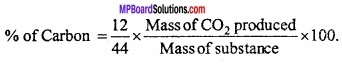Question 3.
What is homologous series? Write its characteristics?
Homologous series is a series of similarly constituted organic compounds in which the members possess the same functional group, have similar or almost similar chemical characteristics, can be represented by the same general formula and the two consecutive members differ by CH2 group in their molecular formulae.

The various members of a particular homologous series are called homologues. A few homologues of alcohol series (containing straight chain alcohols) are as follows: General formula CnH2n+1OHCharacteristics of homologous series:

1. All the members of a series can be represented by the general formula. For example, general formula of alcohol family is CnH2n+1OH.
2. The two successive members of a particular family is differ by – CH2 group or by 14 atomic mass unit (12 + 2 × 1).
3. Different members in a family have common functional group, for example, alcohol family given above.
4. The members of a particular family have almost identical chemical properties and their physical properties such as melting point, boiling point, density, solubility etc. show a proper gradation with the increase in the molecular mass.
5. The members present in a particular series can be prepared almost by similar methods known as the general methods of preparation.

Question 4.
What are primary, secondary, tertiary and quarternary C of organic compound?
Primary carbon atom:
Carbon atom in the organic compound which is linked with only one carbon atom is called primaty (p) or (1°) carbon atom.

Secondary carbon atom:
Secondary carbon atom is that carbon atom which is linked with two more carbon atoms in the compound. It is also represented by (2°) or (s) carbon atom.

Tertiary carbon atom:
The carbon atom which is linked with three more carbon atoms, is called tertiary (3°) or (t) carbon atom.

Quarternary carbon atom:
The carbon atom which is linked with four other carbon atoms, is called quartemary carbon atom. It is denoted by 4° or q.
In the following example primary (p), secondary (s) and tertiary (t) carbon atoms are represented: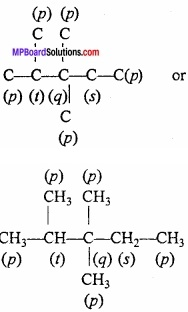Where, p = primary, s = secondary, t = tertiary and q = quartemary.Question 5.
What is resonance? Write its applications?
Sometimes it is found that all the known properties of a compound cannot be explained by one structure and for such compounds we draw two or more structures. (MPBoardSolutions.com) Such structures are called resonating structures or canonical forms or contributing structures and the phenomenon is called resonance or mesomeric effect. This is a permanent effect. This effect is transmitted through the chain. There are two types of resonance or mesomeric effect:

1. + R or + M effect:
A group is said to have +R or +M effect when the displacement of the electron pair is away from it. For example,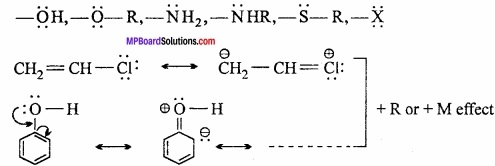2. – R or – M effect:
A group is said to have – R or – M effect when the displacement of the electron patr is towards it. For example,Uses:

1. For determination of structure of benzene.
2. To explain dipole moment.
3. To explain the strength of acids and bases.

Question 6.
What is the reason, that carbon forms large number of compounds?
Or, Explain the special properties of carbon,
Anomalous behaviour of First Element of the Group (Carbon):
Carbon the first member of group – 14 shows an anomalous behaviour i.e., differ from the rest of the members of its family. The main reasons for this difference are:

1. Small atomic and ionic size
2. Higher electronegativity
3. Higher ionisation enthalpy
4. Absence of d – orbital in the valence shell

The main points of difference are:

1. It is an important component of animal kingdom.

2. It is also found in free state in nature.
3. It possesses the property of catenation because C – C bond energy is very high 353.3 kJmol-1.

4. Carbon exist in various allotropic form. Its three crystalline form are diamond, graphite and fullerene.

5. Carbon atom has tendency to form pπ – pπ bond with other carbon atom and also with oxygen, nitrogen, sulphur etc. Due to this, carbon – carbon, carbon – oxygen, carbon – nitrogen, etc. double and triple bonds are possible.

6. Carbon is the only element which forms highly stable open chain, cyclic hydrocarbon and aromatic hydrocarbon with hydrogen.

It is due to its property called catenation. It is the ability of like atoms to link with one another through covalent bonds. This is due to smaller size and higher electronegativity of carbon atom and unique strength of carbon – carbon bond. (MPBoardSolutions.com) Since the bond energy of C – C bond is very large (348 kJ mor1). Carbon forms long straight or branched C – C chains or rings of different size and shape. However, as we move down the group the element – element bond energies decreases rapidly viz C – C (348 kJ mol-1), Si – Si (297 kJ mol-1), Ge – Ge (260 kJ mol-1), Sn – Sn (240 kJ mol-1), Pb – Pb (81 kJ mol-1), and therefore, the tendency for catenation decreases in the order:
C >> Si > Ge = Sn > Pb.

7. Carbon forms three types of oxide, monoxide, dioxide and suboxide. Bond energy of carbon monoxide is highest among diatomic molecules.

8. Carbon atom can link with other metals directly through covalent bonds compound formed are called organometallic compound.Question 7.
Explain metamerism and tautomerism with example?
Metamerism:
The compounds having same molecular formula but different number of carbon atoms (or alkyl group) on either side of the functional group are called metamers and phenomenon is called metamerism.Tautomerism:
This is a special type of functional isomerism in which the isomers differ in the arrangement of atoms but they exist in dynamic equilibrium with each other. For example, acetaldehyde and vinyl alcohol are tautomers.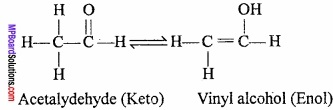Question 8.
What are Nucleophile? Explain with example?
Nucleophiles:
The species having an atom, unshared or ione pair of electron and seeking positive sets are called nucleophiles.
Neutral nucleophiles: NH3, H2O, R – O – R.
Negative nucleophiles: Cl, OH, NH2, CN.Question 9.
What are Electrophiles? Explain with example?
Electrophiles:
The positively charged or neutral species which are deficient of electron and can accept, lone pair of electron are called electrophiles.

Neutral electrophiles:
BF3, AlCl3, FeCl3.

Negative electrophiles:
H3O+, Cl+, NO2+.

Question 10.
How nitrogen is tested in any organic compound by Lassaingen’s method?
Take 2 ml of Sodium extract, add a 2 ml of freshly prepared solution of ferrous sulphate along with 1 – 2ml of NaOH. Heat and then cool the solution. (MPBoardSolutions.com) Green precipitate of Fe(OH)3 is obtained. Add cone. HCl so that green precipitate of ferrous sulphate goes into solution. Then 2 – 3 drops of ferric chloride solution is added. If green or blue colour is obtained then the substance contain nitrogen.Question 11.
How will you test the presence of sulphur in any organic compounds?
Sulphur test:
1. Sodium nitropruside solution is added to the sodium extract if violet colour appears. Confirms the presence of sulphur in given organic compound.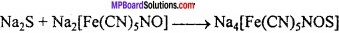2. Sodium extract is acidified with acetic acid and lead acetate solution is added. If black precipitate is obtained confirms the presence of sulphur in organic compound.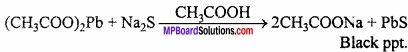Question 12.
A sample of 0.50g of an organic compound was treated according to Kjeldahl’s method. The ammonia evolved was absorbed in SO ml of 0.5M H2SO4. The residual acid required 60 mL of 0.5 M solution of NaOH for neutralization.Find the percentage composition of nitrogen in the compound?
Solution:
Volume of acid taken = 50 ml of 0.5 M H2SO4
= 25 ml of 1.0 M H2SO4
Volume of base used for neutralization of acid
= 60 ml 0.5 M NaOH
= 30 ml 0.1 M NaOH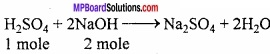∴ 30 ml of 1.0 M NaOH = 15 ml of 1.0 M H2SO4
∴Volume of acid used by ammonia = 25 – 15 = 10 ml
% Amount of Nitogen = image 17
% N = $$\frac{1.4 × 2 × 10}{0.5}$$ = 5 gmN.Question 13.
Steam distillation is useful for which organic compounds? Explain with example?
Steam distillation:
Steam distillation is used to purify those organic compounds which are practically immiscible with water, volatile in steam and has fairly high vapour pressure (low boiling point). In this method, the impure liquid is taken in a heated flask and steam is passed over it with the help of a steam generator (Fig.). (MPBoardSolutions.com) The mixture of steam and the volatile organic compound is condensed and collected in a receiver. From this mixture, water is removed by using separating funnel.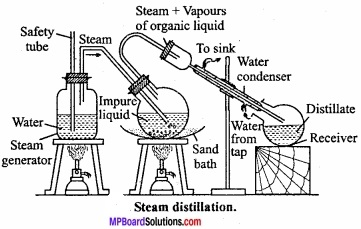During steam distillation, a liquid boils only when the sum of vapour pressure of the liquid (P1) and of water (P2) becomes equal to the atmospheric pressure than its boiling point e.g., a mixture of water and a steam volatile insoluble substance will vaporise close below 373K. (MPBoardSolutions.com) This above technique is used for separating aniline from aniline water mixture and also for separation of p – nitro phenol from p – nitro phenol (o – nitrophenol is steam volatile).

Question 14.
What is the principle of Adsorption chromatography? Explain?
Chromatography:
The process by which different components of a mixture are separated by distributing in stationary or mobile phases on the basis of difference in adsorption abilities on any adsorbent, is called chromatography.

It is also known as column chromatography. It is based on the fact that when solution of mixture comes in contact with some adsorbent, different components of a mixture get adsorbed to different extent on account of difference in power of adsorption.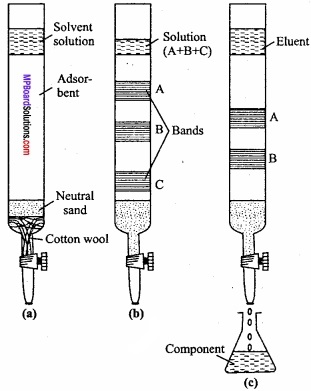Question 15.
0.2 gm of chlorine containing organic substance gave in Carius method 0.2870 gm of AgCl. Determine the percentage of chlorine in the compound?
Weight of organic substance = 0.2 gm.
Weight of AgCl = 0.2870 gm.
Percentage of Chlorine = $$\frac{35.5}{143.5}$$ ×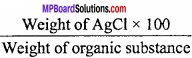= $$\frac{35.5}{143.5}$$ × $$\frac{0.2870 × 100}{0.2}$$
= 35.5%Question 16.
What is principle of vaccum distillation or distillation under reduced pressure 7 Explain with figure?
Distillation under reduced pressure:
Many substances decompose at their boiling points. Hence, they cannot be purified by simple distillation. These compounds are distilled at low temperatures and low pressures. This is known as reduced pressure distillation. (MPBoardSolutions.com) Boiling point of a liquid is the temperature at which the vapour pressure of the liquid is equal to the atmospheric pressure. This means that by lowering the pressure to which a liquid is subjected, the boiling points of the liquid can be lowered. Similarly, if the pressure is increased, the boiling point also increases. This means that a liquid can be made to boil any temperature by varying the pressure.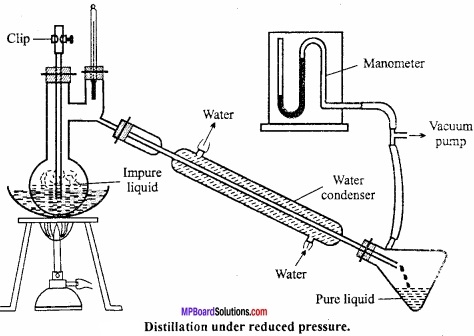As shown in the figure distillation under reduced pressure is carried out in a specially designed flask called Claisen flask. (MPBoardSolutions.com) Pressure in the receiver is reduced by vacuum pump which reduces pressure in distillation flask also and liquid begins to boil at low temperature. For example, glycerol is also distilled under reduced pressure. Its b.p. is 290°C, but using 12 mm pressure it can be distilled at 180°C.

Question 17.
How halogens are detected in organic compound?
AgNO3 Test:
If on adding HNO and AgNO3 in sodium extract, white ppt. comes, then AgCl is present. If the white ppt. is soluble in excess of NH4C1 than Cl is present. On adding dil. HNO3 and AgNO3 in sodium extract, if yellow ppt. appears than bromine and iodine is present.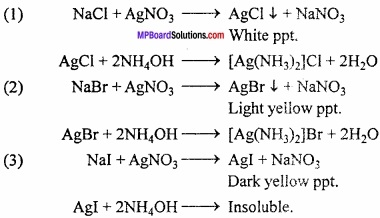Question 18.
Write the difference between Inductive effect and Electrometric effect:
Inductive effect:

1. It is a permanent effect.
2. This arises due to displacement or σ – bond.
3. Partial negative or positive charge develops.
4. Displacement reaction occurs.
5. Always present in molecule.

Electrometric effect:

1. It is temperory effect.
2. Arises due to displacement of or π – bond.
3. Complete positive and negative charge develops.
5. This effect arises due to presence of attacking reagent.

Organic Chemistry: Some Basic Principles and Techniques Long Answer Type Questions:

Question 1.
How halogen is detected in an organic compound?
Estimation of Halogens Carius method:
In this method estimation of halogens (Cl, Br and I) is done. A known weight of organic compound containing halogen is heated with AgNO3 and fuming nitric acid. Carbon, hydrogen and sulphur present in the compound gets oxidized and halogens form AgX (Silver halide).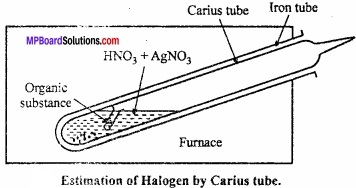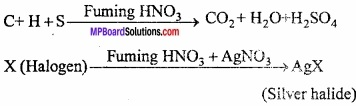Caluculations:
Suppose = W gm
Weight of organic substance = m gm
Weight of silver halide = x gm
Molecular mass of silver halide = (108 + x) gm
Molecular mass of silver halide = x gm
∴ m gm of silver halide contain halogen = $$\frac{x}{(108 + x)}$$ × m gm
W gm of substance contain halogen = $$\frac{x × m}{(108 + x)}$$ gm
∴ 100 gm of organic substance contains = $$\frac{x × m × 100}{(108 + x)}$$ × W
Hence, percentage of halogenQuestion 2.
Write short notes on carbanion?
Carbanion:
Carbanions may be defined as negatively charged ions, in which carbon is having negative charge and it has eight electrons in the valence shell e.g.,Generation of carbanions:
These are mostly generated in the presence of a base by heterolytic cleavage.Types of alkyl Carbions:
Depending on the carbon bearing negative charge carbions may be of threee types,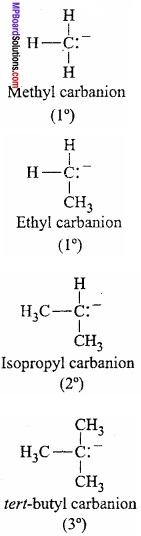Orbital structure of carbanion:
The negatively charged carbon atom in a carbanion is spl hybridized. It is expected to have a tetrahedral geometry. The three hybridized orbitals with one electron each are involved in the σ – bonds with the orbitals of other atom or groups. (MPBoardSolutions.com) The fourth hybridized orbital has overlapped. It is responsible for the negative charge on carbanion and also for the distortion of its geometry. The H actual shape of the carbanion is pyramidal.Stability of carbanion:
The stability of carbanion can be discussed with the help of inductive effect.

Question 3.
What is Inductive effect? Write its uses?
Inductive effect:
In a covalent band between two disimilar atoms having different electro negativities the electron pair does not remain in the centre but gets attracted towards the more elecronegative atoms. (MPBoardSolutions.com) The bond becomes some what polar due to unequal sharing of the electron pair. For example, in the bond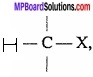if X is more electronegative than C, the electron pair gets attracted towards S. This shifting of electrons develops a partial negative charge denoted by on δ on X and C attains a partial positive charge doneted by δ+. Thus,Now consider a log chain of carbon atoms with a more electronegative element say chlorine attached at one end.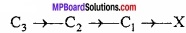The electron pair of the bond between C1 and X gets displaced towards electronegative chlorine atom. This results in developing of partial negative charge on chlorine and partial charge on carbon. This displacement is further transmitted to other carbon atoms of the chain but the magnitude of displacement goes on decreasing with the increases in the distance of the carbon atoms from the chlorine atom as shown below: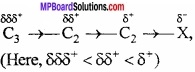Thus, it can be concluded that a polar bond induces polarity in the other covalent bonds in a chain. This type of displacement of electrons is referred to as inductive effect (or I effect) or transmission effect. (MPBoardSolutions.com) Thus, inductive effect may be defined as, the permanent displacement of electrons along the chain of carbon atoms due to presence of polar covalent bond in the chain.

Types of inductive effect:
There are two type of inductive effects:

1. Electron withdrawing inductive effect (- I effect):
If the substantiates attached to the end the carbon chain is electron withdrawing, the effect is called – I effect. The decreasing order or – I effect of some atoms or groups is as follows:2. Electron releasing inductive effect (+1 effect):
If the substantiates attached to the end of the carbon chain is electron releasing, the effect is called +I effect. Alkyl groups are electron releasing in nature. Thus, the decreasing order of +I effect is as follows:Question 4.
Write the differences between electrophilic and nucleophilic reagents?
Electrophilic Reagent:

1. Deficient electrons.
2. Generally electrons are present in valence shell.
3. They are positive ions.
4. Neutral molecules with incomplete octet accept electrons.
5. They are Lewis acids.

Nucleophilic Reagents:

1. More electron present.
2. Generally 8 electrons are present in valence shell.
3. Negatively charged ion.
4. They are electron pair donor.
5. They are Lewis base.

Question 5.
Explain the Column chromatography technique for purification of organic compounds?
Column chromatography:
It is based on the fact that when solution of mixture comes in contact with some adsorbent, different components of a mixture get adsorbed to different extent on account of difference in power of adsorption.

Column chromatography:
There are three steps of column chromatography:

A long tube like burette is filled with a paste of a suitable adsorbent like activated Solvent Separation Continue elution magnesia, alumina, gypsum, silica gel, kieselguhr, etc. in a suitable organic solvent. The paste is prepared in that solvent in which the solution of the mixture to be separated, is prepared. When adsorbent is set, solvent is allowed to flow down.The substance or the mixture to be adsorbed is dissolved in least quantity of the nonpolar solvent like petroleum ether, benzene, etc. The solution is allowed to flow down the column. The different components of mixture get adsorbed in different parts of column. (MPBoardSolutions.com) The compound which is strongly adsorbed remains in the upper part of the column forming a band. Each component of mixture forms a separate band at a definite place. The coloured band formed are seen clearly. In case the bands are not seen due to being colourless, they are made visible by using a suitable indicator.

3. Elution:
In this process the adsorbed substance is extracted by a suitable solvent. The solvent used for this purpose is called eluent and the process is called elution. The solvents are used in the order of increasing polarity. Solvent in the increasing order of polarity are petroleum ether, petroleum ether containing benzene, alcohol with ether and pure ether.

These solvents are added one after the other. The substance which has been least adsorbed gets extracted with a solvent which is least polar while the component which has been adsorbed more strongly than others, is extracted by more polar solvent like alcohol. (MPBoardSolutions.com) By this way various components of mixture can be separated on several steps.

The various components can be separated from solvent by distillation or by using separating funnel. This technique is employed for separation of complex compounds like vitamins and hormones. The method is also employed for determination of purity of substance.Question 6.
Write the IUPAC name of following compounds: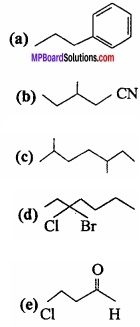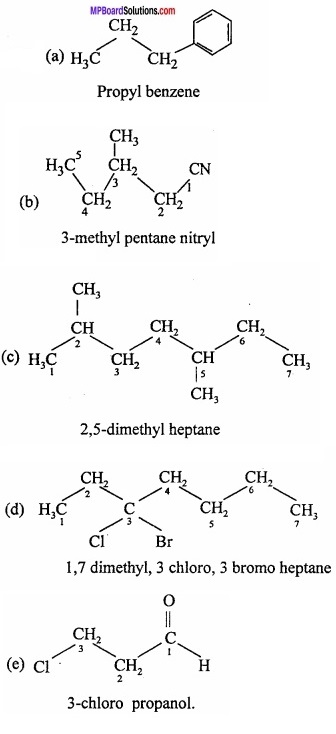Question 7.
Difference betweeen Aliphatic compound and Aromatic compound?
Aliphatic compound:

1. These are open chain compound.
2. Generally C – C bond present.
3. Highly reactive.
4. Halogenation, nitration, sulphonation easily not occur.
5. Combustion energy high.
6. – OH group is neutral.

Aromatic compound:

1. These are closed chain compound.
2. Conjugated single and double bond present.
3. Less reactive.
4. Halogenation, nitration, sulphonation occur.
5. Combustion energy low.
6. – OH group is acidic in nature.

Question 8.
Explain the Duma’s method of determination of nitrogen in organic compound?
Duma’s method:
This method can be used for estimation of nitrogen in all types of nitrogenous compounds. A known amount of nitrogen containing organic compound is heated with cupric oxide (CuO) in an atmosphere of CO2. C and H2O are oxidised to CO2 and H2O while N2 gas is set free.
C + 2Cuo → CO2 + 2Cuo
H2 + Cuo → H2O + Cu (in organic substance)
Nitrogen + CuO → N2 + Some amounts of some oxides of N2
A general equation for nitrogen containing compound is given below:
CxHyNz + (2x + $$\frac{y}{2}$$) CuO → xCO2 + $$\frac{y}{2}$$ H2O + $$\frac{z}{2}$$ N2 + (2x + $$\frac{y}{2}$$) Cu
If sulphur is present in the organic compound. It is converted into S02. During the above reaction, some oxides of nitrogen also be formed. (MPBoardSolutions.com) Therefore, the gaseous mixture is passed over heated reduced copper gauze which converts oxides of nitrogen back to nitrogen.
2NO + 2Cu → 2CuO + N2
2NO2 + 4Cu → 4CuO + N2
The gaseous mixture containing CO2, H2O, SO2 and N2 is collected in a graduated nitrometer containing KOH solution. Water vapours are condensed whereas CO2 and SO2 are absorbed by KOH solution. Nitrogen is collected in the upper part of nitrometer. Volume of nitrogen is noted at room temperature and pressure.

Apparatus:
The main part of apparatus is combustion tube. It is a long tube, open at both the ends. The tube is packed with

1. Oxidized copper gauze which prevents backward diffusion of gases produced during combustion
2. CuO containing weighed amount of organic compound
3. Coarse CuO which oxidises the organic compound into CO2, H2O, SO2 etc.,
4. A reduced copper oxide i.e., copper which converts oxides of nitrogen (NO, NO2 etc.) back to nitrogen.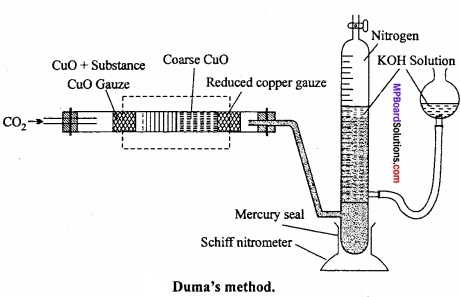Oxides of nitrogen when passed over hot reduced copper gauze change to nitrogen by losing their oxygen.
Oxides of nitrogen + Cu → N2 + CuO

The nitrogen set free is passed through SchifFs nitrometer filled with 40% solution of caustic potash. Caustic potash solution absorbs CO2 while water formed gets condensed.
After the completion of combustion again CO2 is passed through combustion tube to drive out all the remaining nitrogen gas to nitrometer.

The apparatus is cooled. Reservoir bulb of nitrometer is raised so that level of KOH becomes the same in reservoir bulb and nitrometer which means pressure becomes equal to atmospheric pressure. (MPBoardSolutions.com) Now, the volume of nitrogen in nitrometer is noted. Temperature of reaction and atmospheric pressure is noted from barometer. Aqueous tension at that temperature is noted from tables.

Observations and calculation:
Let,

1. Weight of organic substance = W gm
2. Volume of moist N = V ml
3. Temperature = t°C
4. Atmospheric pressure = P mm of Hg
5. Aqueous tension at t°C = p mm
6. Pressure of dry nitrogen = (P – p) mm

Calculation of volume of nitrogen at N.T.P.:Question 9.
In an organic compound C = 40%, O = 53.34 % and H = 6.66%. If the vapour density is 30. Determine the molecular formula?
Or
In an organic compound A, C = 40% and H = 6.66%. The vapour density of A is 30. It turns blue litmus red and can react with ash. When its sodium salt is heated with soda lime, the first member of paraffin series obtained. What is A?
Solution:
C = 40%, H = 6.66%
O = 100 – [40 + 6.66] = 100 – 46.66 = 53.34%
In organic compound A: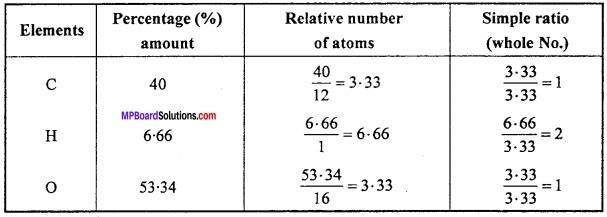Empirical formula of A = H2O
Empirical formula mass = 12 + 2 + 16 = 30
Molecular mass = 2 × Vapour density
= 2 × 30 = 60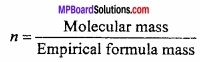= $$\frac{60}{30}$$ = 2
∴ Molecular formula = (CH2O)2
= C2H4O2 or CH3COOH.
It is CH3COOHQuestion 10.
Explain the following with example:

1. Simple distillation
2. Chromatography
3. Crystallization.

1. Simple distillation:
This method is employed for the purification of those liquids which boil without decomposition and are associated with non – volatile impurities. Liquids which have a difference of 30 – 40°C in their boiling points are purified by this method. On heating the mixture, vapours of pure substance are formed which condenses as they pass through the air or water condenser. (MPBoardSolutions.com) The pure liquid collects in the receiver while the non – volatile impurities are left behind in the flask. Some glass beads are also added to the distillation flask to avoid bumping.2. Chromatography:
The process by which different components of a mixture are separated by distributing in stationary or mobile phases on the basis of difference in adsorption abilities on any adsorbent, is called chromatography.It is also known as column chromatography. It is based on the fact that when solution of mixture comes in contact with some adsorbent, different components of a mixture get adsorbed to different extent on account of difference in power of adsorption.

The mixture to be separated and purified is first dissolved in a suitable nonpolar organic solvent like petroleum ether, benzene, chloroform, alcohol, etc. This solution is allowed to flow down an absorption column.

For using this technique there should be proper adsorption column. Adsorbents like activated magnesia, alumina, calcium carbonate, gypsum, etc. is filled in a hard vertical tube (adsorbent column.).

3. Crystallization:
The method by which crystals of a substance can be made is called crystallization. Solids can be separated and purified by crystallization. e.g., nitre, alum, copper sulphate, etc. Some impure solids which differ in solubility in the same solvent, their separations can be achieved by fractional crystallization. (MPBoardSolutions.com) “If two or more components of a mixture which differ in their solubilities are dissolved in a solvent in which their solubilities slightly differ, they can be separated by fractional crystallization.”

Suppose, two solids A and B are dissolved in a solvent. If solubility of A is less as compared to B, then first saturated solution of the mixture is prepared and is allowed to cool. During crystallization first less soluble substance A will crystallize out. (MPBoardSolutions.com) It is separated by filtration. After this crystals of more soluble substance B will separate out. By this technique, crystals of both can be separated. The substances so separated are further crystallized many times to get pure substances.Question 11.
Write the IUPAC name of following compounds:

(a) CH3CH = C(CH3)2
(b) CH2 = CH – C = C – CH2
(c)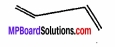(d)(e)